I found this image here.

You can be sure that you’re going to encounter exponents on the SAT. Hopefully, once you’ve been through this post, you’ll look forward to the opportunity to spank these questions like they deserve. Look at them, mocking you. They’re totally asking for it. Show no mercy.

The Basic Exponent Rules
• Anything to the first power equals itself:
y1 = y
• Anything raised to the zero power equals 1:
m0 = 1
• Remember that a regular old integer exponent means something very simple:
x4 = xxxx. Don’t be ashamed to do this when you’re stuck. You’ll be amazed how often this will unstick you.
• When you multiply like bases, you add their exponents:
p3p5 = p3+5 = p8. Why? See the bullet above.
• When you divide like bases, you subtract the exponents:
r9/r4 = r9-4 = r5. Again, why is this true? Think about it. Does it blow your mind?
• When you raise an exponent to another power, you multiply the exponents:
(z3)7 = z21. Why is that?
• When you’re adding or subtracting like bases, you can’t do JACK:
y3 + y4y3y4. There’s no simplifying (although you might decide to factor a y3 out depending on where you’re trying to go — remember this for practice problem #18 below). If you make the mistake of adding these exponents, people will laugh at you in public places.

Before we go further, let’s make sure you’ve got this. Try simplifying the following. Hold your mouse over the questions when you’re done, and the answers will reveal themselves, as though they were genies and you Aladdin. Don’t click, just hover.

• t9t2 =
• 3n/32 =
• (xy3)(x5y) =
• (8m)2 =
• r16r12 =
Fractional and Negative Exponents

Honestly, these appear very rarely on the SAT. I’m including them for completeness’s sake, but don’t sweat these too hard.

• Fractional exponents (to convert the exponent to radical form):
• The numerator is the power.
• The denominator is the root.
• In other words: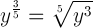• Negative exponents
• A negative exponent signifies the reciprocal of the corresponding positive exponent.
• In other words, the base is on the wrong side of the fraction line.
• In other words: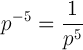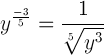What’s nice is that even though these rules might be a bit more confusing, fractional and negative exponents follow the same rules as integer exponents. Stare at these for a minute to see what I mean: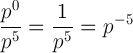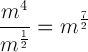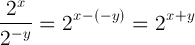Try some practice questions now, bro!

You need to be registered and logged in to take this quiz. Log in or Register